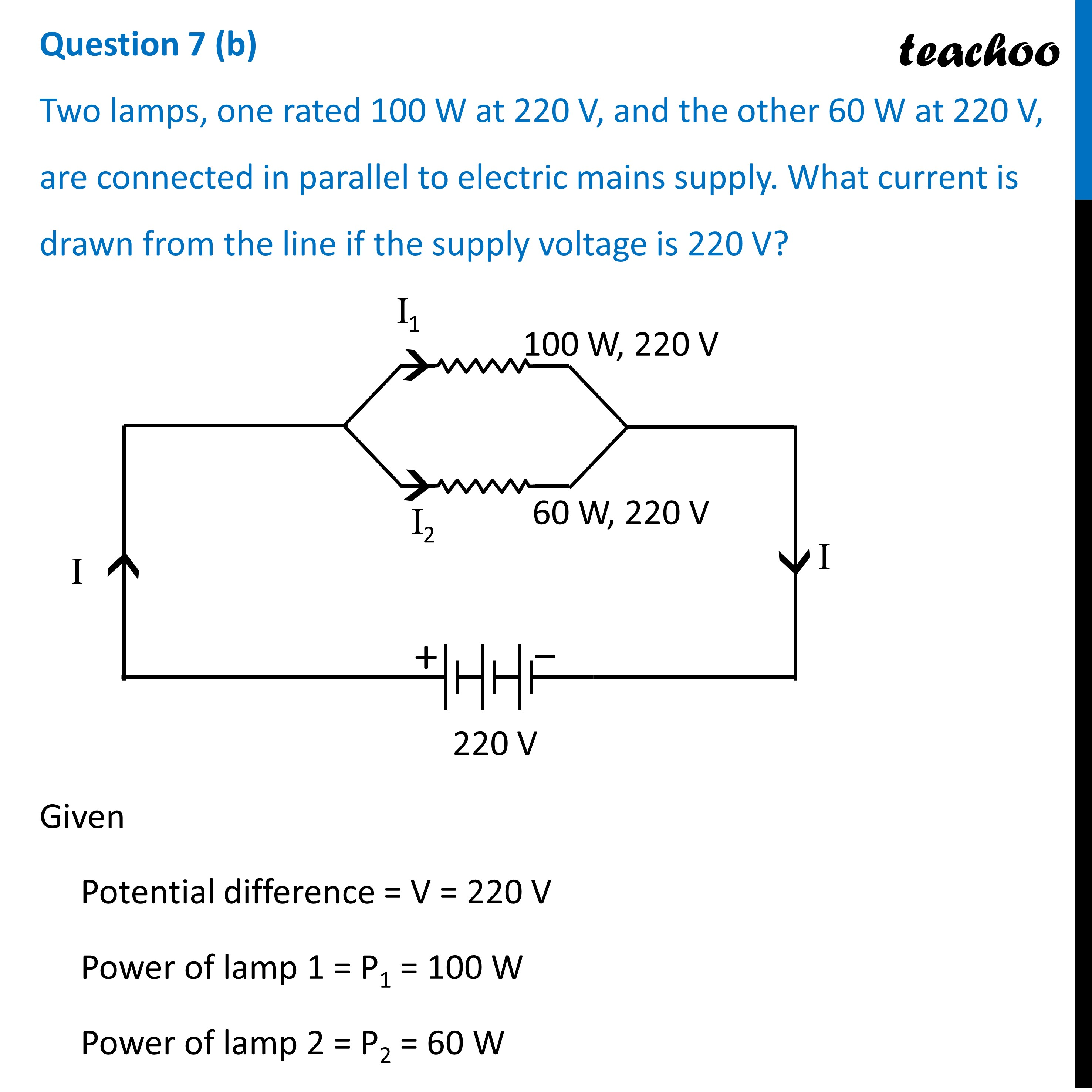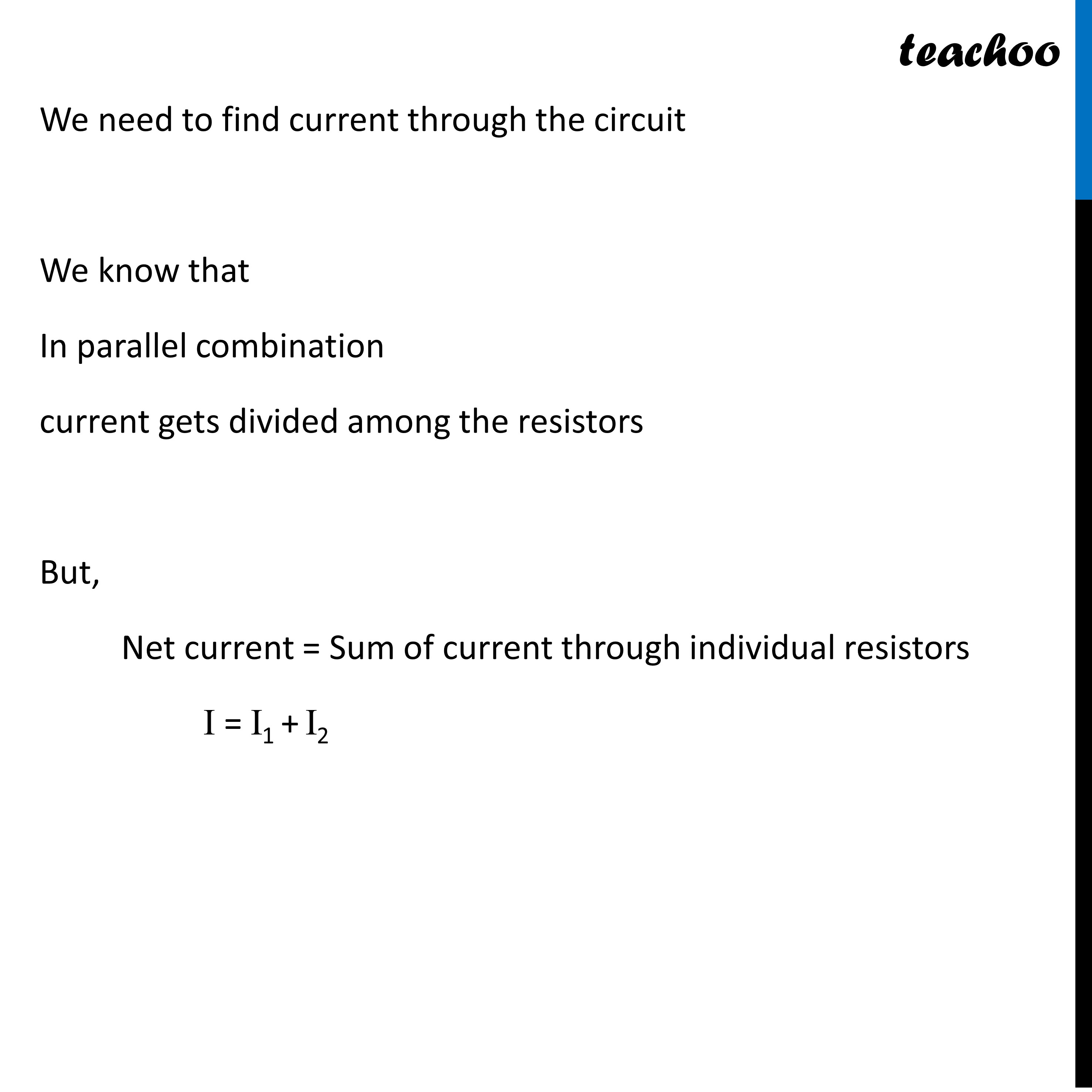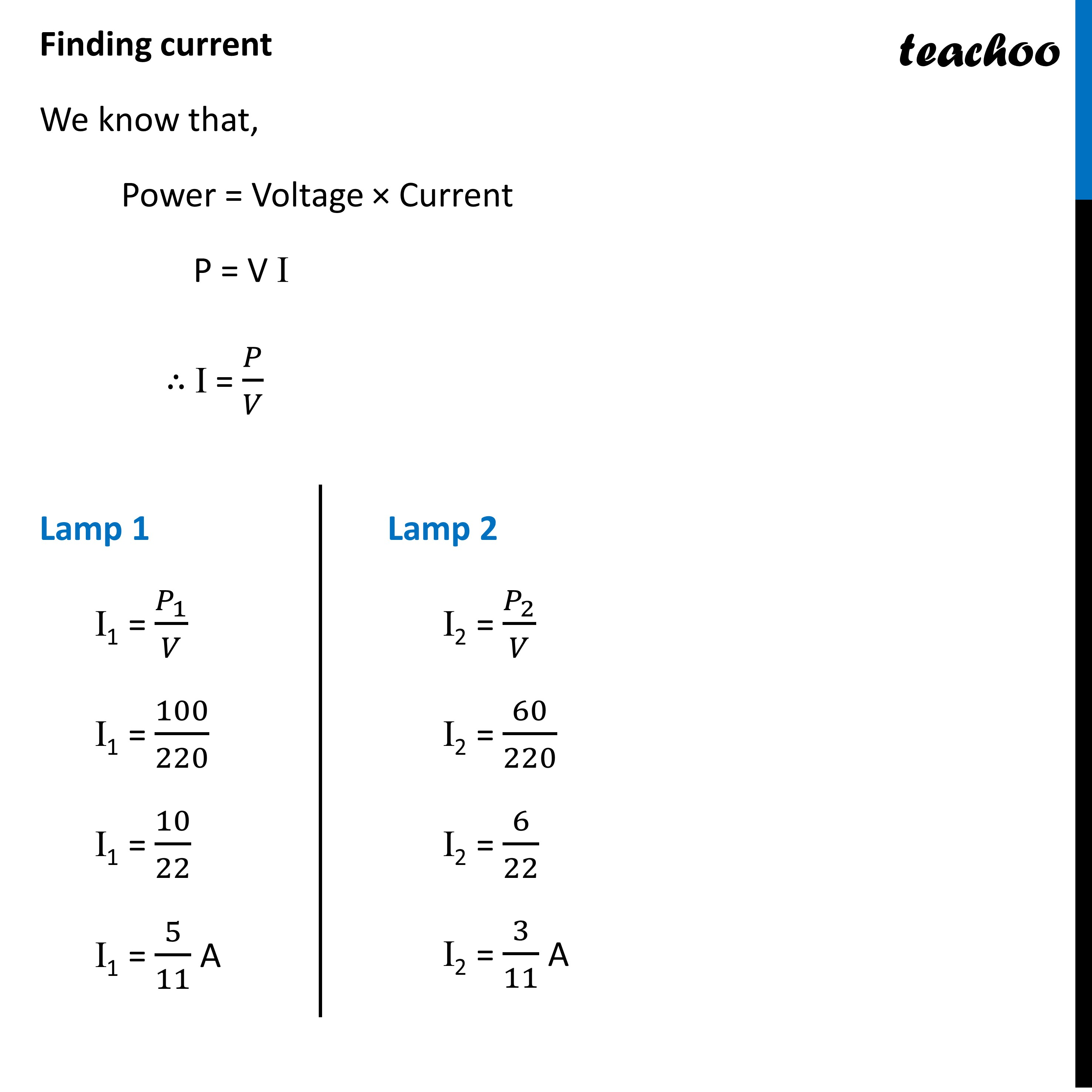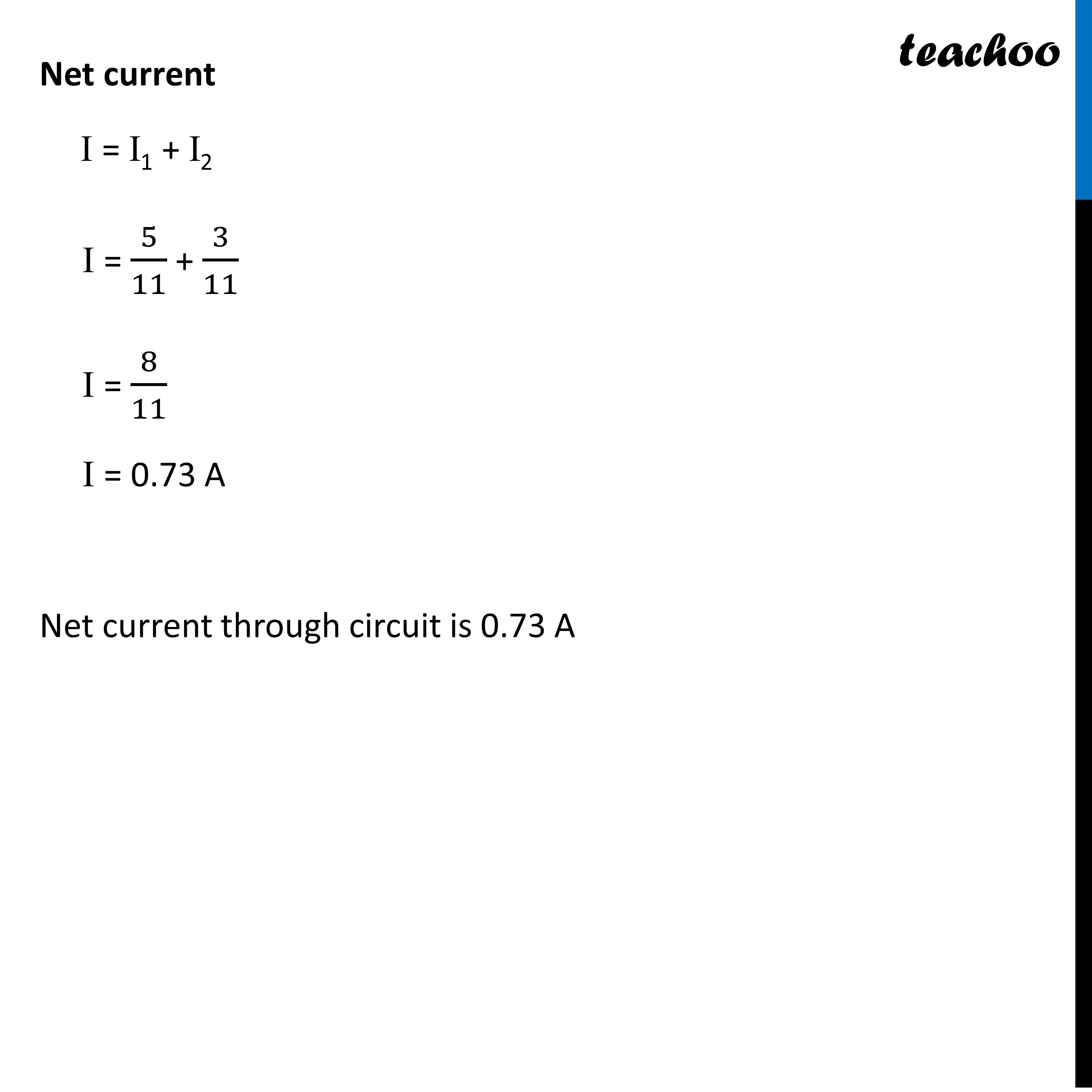Past Year - 3 Mark Questions

Class 10
Chapter 12 Class 10 - Electricity

## (b) Two lamps one rated 100 W 220 V, and the other 60 W 220 V, are connected in parallel to electric mains supply. Find the currents drawn by two bulbs from the line, if the supply voltage is 220 V.

(a)

Joule’s law of heating states that the heat produced in a conductor is directly proportional to

(i) the square of  the current I through it,

(ii) its resistance R and

(iii) the time t , for which current is passed.

Therefore, the mathematical expression of Joule’s law of heating is:

H = I 2 Rt

Where,

• H = Produced Heat
• I = Current flowing through the device
• t = Time of current flow
• R = Resistance of the appliance

(b)Learn in your speed, with individual attention - Teachoo Maths 1-on-1 Class

### Transcript

Question 7 (b) Two lamps, one rated 100 W at 220 V, and the other 60 W at 220 V, are connected in parallel to electric mains supply. What current is drawn from the line if the supply voltage is 220 V?Given Potential difference = V = 220 V Power of lamp 1 = P1 = 100 W Power of lamp 2 = P2 = 60 W We need to find current through the circuit We know that In parallel combination current gets divided among the resistors But, Net current = Sum of current through individual resistors I = I1 + I2 Finding current We know that, Power = Voltage × Current P = V I ∴ I = 𝑃/𝑉 Lamp 1 I1 = 𝑃_1/𝑉 I1 = 100/220 I1 = 10/22 I1 = 5/11 A Lamp 2 I2 = 𝑃_2/𝑉 I2 = 60/220 I2 = 6/22 I2 = 3/11 ANet current I = I1 + I2 I = 5/11 + 3/11 I = 8/11 I = 0.73 A Net current through circuit is 0.73 A